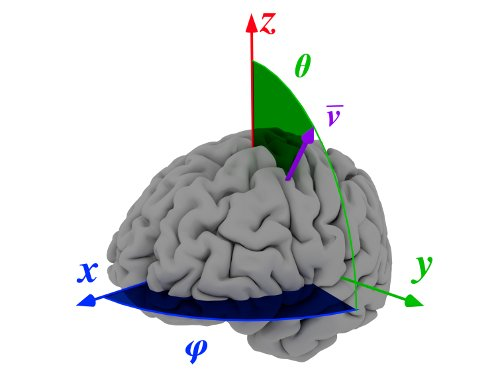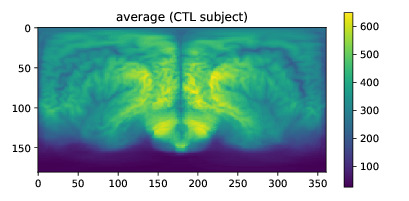# Quickstart on mapBrain¶

mapBrain is a library used to perform the Spherical Brain Mapping on neuroimaging.

## Spherical Brain Mapping¶

The Spherical Brain Mapping (SBM) is a feature extraction and visualization framework intended to map the internal structures and features of the brain onto a 2D image that summarizes all this information.SBM allows three-to-two dimension mapping by means of some statistical measures. The system is based on a conversion from 3D spherical to 2D rectangular coordinates. For each spherical coordinate pair $$(\theta,\varphi)$$, a vector $$\mathbf{v}_{(\theta,\varphi)}$$ oriented in that direction and starting at the defined origin (usually the Anterior Commisure), is defined. This vector creates a set of voxels $$V_{(\theta,\varphi)}$$ that contains the intensities of all voxels crossed by $$\mathbf{v}_{(\theta,\varphi)}$$.

From $$V_{(\theta,\varphi)}$$, a number of SBM measures can be computed, including including statistical values (average, entropy, kurtosis) and morphological values (tissue thickness, distance to the central point, number of non-zero blocks). These values conform a two-dimensional image that can be computationally and visually analysed.

## Installation¶

mapBrain is now available via pypi and can be installed directly from:

pip install mapBrain


cd /path/to/uncompressed/mapBrain/
python setup.py install


## Quick usage¶

mapBrain transforms 3D brain images in 2D maps showing texture and statistical information. To use it, import the mapBrain library and create an object of SphericalBrainMapping class:

import mapBrain
sbm = mapBrain.SphericalBrainMapping()


Imaging information must be in numpy.ndarray format, so you can load the images using, e.g., nibabel as:

import nibabel as nib


Once the image has been load, extract the relevant information as:

imarray = image.get_data()
affine = image.affine


If no origin is specified, mapBrain directly uses the geometrical center of the image. To specify the Anterior Commisure as origin, work with the image affine and extract this position on the array:

posAC = image.affine[:,3]/affine.diagonal()


The position may vary depending on the registration procedure used. To quickly generate and visualize a SBM measure of an image, do:

aux = sbm.doSBM(imarray, origin=-posAC, measure='average', show=True)


That should output a window (if not, use plt.show() of matplotlib.pyplot) with an average map just like this: